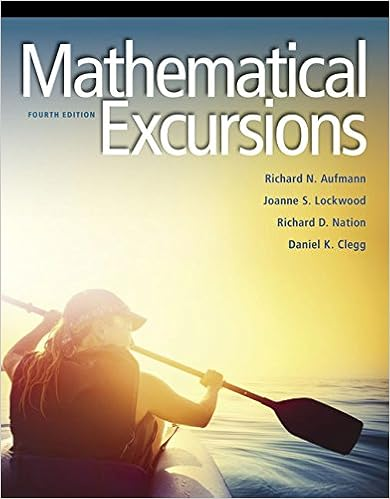# In one way analysis of cholesterol data cohort is the

• Notes
• studybuddy929
• 256

This preview shows page 124 - 129 out of 256 pages.

##### We have textbook solutions for you!
The document you are viewing contains questions related to this textbook.The document you are viewing contains questions related to this textbook.
Chapter 13 / Exercise 02
Mathematical Excursions
AufmannExpert Verified
In one-way analysis of cholesterol data, COHORT is the only factor. This factor can be broken down into two factors in a two-way analysis: AGE (factor A) and GENDER (factor B). Definition : If there are observations at all combinations of all factors, the design is complete , otherwise it is incomplete .
##### We have textbook solutions for you!
The document you are viewing contains questions related to this textbook.The document you are viewing contains questions related to this textbook.
Chapter 13 / Exercise 02
Mathematical Excursions
AufmannExpert Verified
Osborne, ST512 124 Exercise 1. Estimate the mean difference in cholesterol between young men and young women. 2. Estimate the mean difference between old men and old women. 3. Estimate the mean difference between men and women. 4. Estimate the mean difference between older and younger folks. 5. Estimate the mean difference between the differences estimated in 1. and 2. 6. Provide standard errors for all of these estimated contrasts 7. Specify the vectors defining these contrasts. For example, the first contrast of cohort means can be written θ 1 = ( - 1 , 1 , 0 , 0) 0 μ 1 μ 2 μ 3 μ 4 = μ 2 - μ 1
Osborne, ST512 125 Consider the following contrasts of the cohort cholesterol means in the population: θ 3 = ( - 1 , 1 , - 1 , 1) 0 μ θ 4 = ( - 1 , - 1 , 1 , 1) 0 μ θ 5 = ( - 1 , 1 , 1 , - 1) 0 μ Q: Are these contrasts orthogonal? Q: True/False: SS ( ˆ θ 3 ) + SS ( ˆ θ 4 ) + SS ( ˆ θ 5 ) = SS [ Trt ] Another exercise: 1. Compute the sums of squares for the estimated contrasts in 3., 4. and 5. using the exercise just completed and the fact that if ˆ θ = c i ¯ y i + then SS [ ˆ θ ] = ˆ θ 2 c 2 i n i . 2. Formulate a test of H 0 : θ i = 0 for each of these three contrasts. Obtain the F -ratio for each of these tests. 3. Obtain the α = 0 . 05 critical region for each test. Compare the observed F -ratios to critical value and draw conclusions about (a) an age effect (b) a gender effect (c) an age × gender interaction
Osborne, ST512 126 Types of effects Two-way ANOVA model for the cholesterol measurements: Y ijk = μ + α i + β j + ( αβ ) ij + E ijk i = 1 , 2 = a and j = 1 , 2 = b and k = 1 , 2 , . . . , 7 = n. E ijk iid N (0 , σ 2 ). Parameter constraints: i α i = j β j = 0 and i ( αβ ) ij 0 for each j and j ( αβ ) ij 0 for each i . Factor A: AGE has a = 2 levels - A 1 : younger and A 2 : older Factor B: GENDER has b = 2 levels - B 1 : female and B 2 : male Three kinds of effects in 2 × 2 designs: 1. Simple effects are simple contrasts. μ ( A 1 B ) = μ II - μ I - simple effect of gender for young folks. μ ( AB 1 ) = μ III - μ I - simple effect of age for women 2. Interaction effects are differences of simple effects: μ ( AB ) = μ ( AB 2 ) - μ ( AB 1 ) = ( μ IV - μ II - ( μ III - μ I )) - difference between simple age effects for men and women - difference between simple gender effects for old and young folks - interaction effect of AGE and GENDER. 3. Main effects are averages or sums of simple effects μ ( A ) = 1 2 ( μ ( AB 1 ) + μ ( AB 2 )) μ ( B ) = 1 2 ( μ ( A 1 B ) + μ ( A 2 B )) Exercise: Classify the contrasts in the last exercise as simple, inter- action or main effects.
Osborne, ST512 127 Partitioning the treatment SS into t - 1 orthogonal components 12281 = SS [ Trt ] = SS [ ˆ θ 3 ] + SS [ ˆ θ 4 ] + SS [ ˆ θ 5 ] = 5103 + 6121 + 1056 ( a - 1)( b - 1) df for AB interaction ( a - 1) df for main effect of A ( b - 1) df for main effect of B F test for interaction effect To test for interaction, H 0 : ( αβ ) 11 = ( αβ ) 12 = ( αβ ) 21 = ( αβ ) 22 = 0 vs.
•••